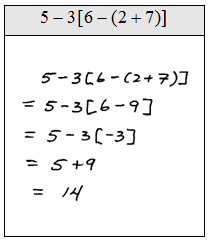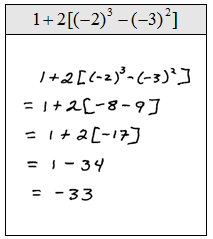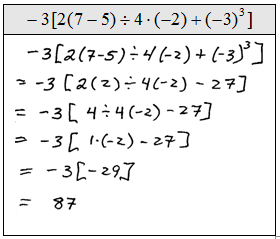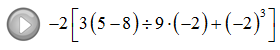## Algebra

### Order of Operations

When several operations are to be applied within a calculation, we must follow a specific order to ensure a single correct result.

Order of Operations:
1. Perform all calculations within the innermost Parentheses first.
2. Evaluate Exponent expressions.
3. Apply the Multiplication and Division operations from left to right.
4. Lastly, work all Addition and Subtraction operations from left to right.
Caution:  Please do not dismiss the fact that multiplication and division should be worked from left to right.  Many standardized exams will test you on this fact.  The following example illustrates the problem.Order of Operations - The Basics

Simplify.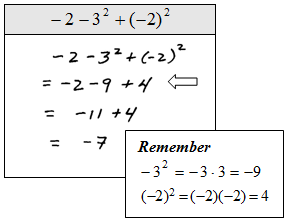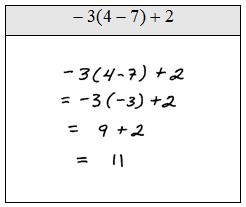Order of operation problems get a bit more tedious when fractions are involved. Remember that when adding or subtracting fractions you need to first find the equivalent fractions with a common denominator. Multiplication does not require a common denominator.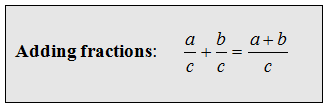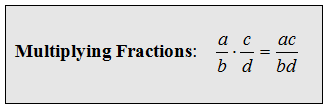Simplify.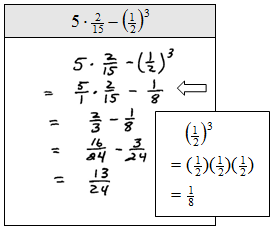The Order of Operations

We will see that some of the problems have different looking parentheses { [ ( ) ] }, treat them the same and just remember to perform the innermost parentheses first.  Some problems may involve an absolute value, in which case you will need to apply the innermost absolute value first as you would if it were a parentheses.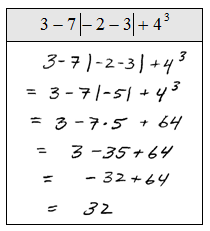To avoid these unnecessary mistakes, work one operation at a time and for each step rewrite everything.  This may seem like a lot of work but it really helps avoid errors.

Simplify.